# Samacheer Kalvi Books: Tamilnadu State Board Text Books Solutions

## Samacheer Kalvi 10th Science Chapter 4 Electricity Notes PDF Download: Tamil Nadu STD 10th Science Chapter 4 Electricity NotesSamacheer Kalvi 10th Science Chapter 4 Electricity Notes PDF Download: Tamil Nadu STD 10th Science Chapter 4 Electricity Notes

We bring to you specially curated Samacheer Kalvi 10th Science Chapter 4 Electricity Notes PDF which have been prepared by our subject experts after carefully following the trend of the exam in the last few years. The notes will not only serve for revision purposes, but also will have several cuts and easy methods to go about a difficult problem.

 Board Tamilnadu Board Study Material Notes Class Samacheer Kalvi 10th Science Subject 10th Science Chapter Chapter 4 Electricity Format PDF Provider Samacheer Kalvi Books

## How to Download Samacheer Kalvi 10th Science Chapter 4 Electricity Notes PDFs?

2. Click on the Samacheer Kalvi 10th Science Notes PDF.
3. Look for your preferred subject.
4. Now download the Samacheer Kalvi 10th Science Chapter 4 Electricity notes PDF.

## Download Samacheer Kalvi 10th Science Chapter 4 Electricity Chapterwise Notes PDF

### Samacheer Kalvi 10th Science Electricity Textual Solved Problems

10th Science Electricity Lesson Book Back Answers Question 1.
A charge of 12 coulomb flows through a bulb in 5 seconds. What is the current through the bulb?
Solution:
Charge Q = 12 C, Time t = 5s. Therefore,
Current I=Qt=125=2.4A.

Electricity Class 10 Book Back Answers Question 2.
The work done in moving a charge of 10 C across two points in a circuit is 100 J. What is the potential difference between the points?
Solution:
Charge Q = 10 C, Work Done W = 100 J
Potential Difference V=WQ=10010
Therefore, V = 10 volt.

Electricity Book Back Answers Question 3.
Calculate the resistance of a conductor through which a current of 2 A passes, when the potential difference between its ends is 30 V.
Solution:
Current through the conductor I = 2 A,
Potential Difference V = 30 V
From Ohm’s Law: R = VI
Therefore, R = 302 = 15 Ω.

10th Science 1 Chapter 4 Question 4.
The resistance of a wire of length 10 m is 2 ohm. If the area of cross-section of the wire is 2 × 10-7 m2, determine its

1. Resistivity
2. Conductance
3. Conductivity

Solution:
Given: Length, L = 10 m, Resistance, R = 2 ohm
and Area, A = 2 × 10-7 m2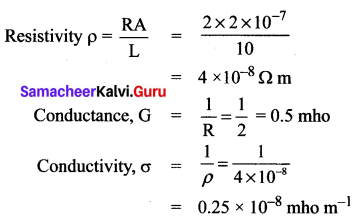10th Science Electricity Lesson Question 5.
Three resistors of resistances 5 ohms, 3 ohms and 2 ohms are connected in series with 10 V battery. Calculate their effective resistance and the current flowing through the circuit.
Solution:
R1 = 5 Ω, R2 = 3 Ω, R3 = 2 Ω, V = 10 V
RS = R1 + R2 + R3 = 5 + 3 + 2 = 10 Ω
The current, I=VRs=1010=1A.

Numericals On Joule’s Law Of Heating Class 10 Question 6.
An electric heater of resistance 5 Ω is connected to an electric source. If a current of 6 A flows through the heater, then find the amount of heat produced in 5 minutes.
Solution:
Given resistance R = 5 Ω, Current I = 6 A,
Time t = 5 minutes = 5 × 60 s = 300 s
Amount of heat produced, H = I2Rt = 62 × 5 × 300 = 54000 J
Hence, H = 54000 J.

Numericals On Ohm’s Law Class 10 Question 7.
Two bulbs are having the ratings as 60 W, 220 V and 40 W, 220 V respectively. Which one has a greater resistance?
Solution:
Electric power P=V2R
For the same value of V, R is inversely proportional to P.
Therefore, the lesser the power, the greater the resistance
Hence, the bulb with 40 W, 220 V rating has greater resistance.

Class 10 Electricity Book Back Answers Question 8.
Calculate the current and the resistance of a 100 W, 200 V electric bulb in an electric circuit.
Solution:
Power P = 100 W and Voltage V = 200 V
Power P = VI
So, Current, I = PV = 100200 = 0.5 A
Resistance, R = VI = 2000.5 = 400 Ω.

Samacheer Kalvi Guru 10th Science Question 9.
In the circuit diagram is given below resistors R1, R2 and R3 of 5 Ω, 10 Ω and 20 Ω respectively are connected as shown in fig.
Calculate:
(A) Current through each resistor
(B) The total current in the circuit
(C) The total resistance in the circuit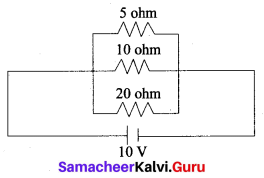Solution:
(A) Since the resistors are connected in parallel, the potential difference across each resistor is same (i.e. V = 10 V)
Therefore, the current through R1 is, I1=VR1=105=2A
Current through R2, I2=VR2=1010=1A
Current through R3, I3=VR1020=0.5A

(B) Total current in the circuit, I = I1 + I2 + I3 = 2 + 1 + 0.5 = 3.5 A

(C) Total resistance in the circuit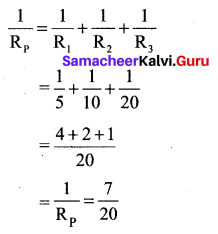Hence, Rp=207=2.857Ω.

Question 10.
Three resistors of 1 Ω, 2 Ω and 4 Ω are connected in parallel in a circuit. If a 1 Ω resistor draws a current of 1 A, find the current through the other two resistors.
Solution:
R1 = 1 Ω, R2 = 2 Ω, R3 = 4 Ω, Current I1 = 1 A
The potential difference across the 1 Ω resistor = I1R1 = 1 × 1 = 1 V
Since, the resistors are connected in parallel in the circuit, the same potential difference will exist across the other resistors also.
So, the current in the 2 Ω resistor, VR2=12=0.5A
Similarly, the current in the 4 Ω resistor, VR3=14=0.25A.

### Samacheer Kalvi 10th Science Electricity Textual Evaluation

Question 1.
Which of the following is correct?
(a) Rate of change of charge is electrical power.
(b) Rate of change of charge is current.
(c) Rate of change in energy is current.
(d) Rate of change of current is a charge.
(b) Rate of change of charge is current

Question 2.
SI unit of resistance is ______.
(a) mho
(b) joule
(c) ohm
(d) ohmmeter.
(c) ohm

Question 3.
In a simple circuit, why does the bulb glow when you close the switch?
(a) The switch produces electricity.
(b) Closing the switch completes the circuit.
(c) Closing the switch breaks the circuit.
(d) The bulb is getting charged.
(d) The bulb is getting charged.

Question 4.
Kilowatt-hour is the unit of _______.
(a) resistivity
(b) conductivity
(c) electrical energy
(d) electrical power.
(c) electrical energy

II. Fill in the blanks

Question 1.
When a circuit is open, ______ cannot pass through it.
Current.

Question 2.
The ratio of the potential difference to the current is known as ______.
Ohm’s law.

Question 3.
The wiring in a house consists of ______ circuits.
domestic electric

Question 4.
The power of an electric device is a product of _____ and ______.
electric current, the potential difference.

Question 5.
LED stands for ______.
Light Emitting Diode.

III. State whether the following statements are true or false: If false correct the statement.

Question 1.
Ohm’s law states the relationship between power and voltage.
False.
Correct Statement: Ohm’s law states the relationship between potential difference and current.

Question 2.
MCB is used to protect household electrical appliances.
True.

Question 3.
The SI unit for electric current is the coulomb.
False.
Correct Statement: The SI unit of electric current is Ampere.

Question 4.
One unit of electrical energy consumed is equal to 1000 kilowatt-hour.
True.

Question 5.
The effective resistance of three resistors connected in series is lesser than the lowest of the individual resistances.
False.
Correct Statement: The effective resistance of three resistors connected in series is greater than the highest of the individual resistance.

IV. Match the items in column-I to the items in column-II:

Question 1.

 1. Electric current (a) Volt 2. Potential difference (b) Ohmmeter 3. Specific resistance (c) Watt 4. Electrical power (d) Joule 5. Electrical energy (e) Ampere

1. (e) Ampere
2. (a) Volt
3. (b) Ohmmeter
4. (c)Watt
5. (d) Joule

V. Assertion and Reason Type Questions

Mark the correct choice as
(a) If both the assertion and the reason are true and the reason is the correct explanation of the assertion.
(b) If both the assertion and the reason are true, but the reason is not the correct explanation of the assertion.
(c) If the assertion is true, but the reason is false.
(d) If the assertion is false, but the reason is true.

Question 1.
Assertion: Electric appliances with a metallic body have three wire connections.
Reason: Three – pin connections reduce heating of the connecting wires
(c) If the assertion is true, but the reason is false.
Correct Reason: Three – pin connections to protect the electrical shocking. Because appliances carry high electric current.

Question 2.
Assertion: In a simple battery circuit the point of highest potential is the positive terminal of the battery.
Reason: The current flows towards the point of the highest potential.
(c) If the assertion is true, but the reason is false.
Correct Reason: The current flows towards the points of the lower potential.

Question 3.
Assertion: LED bulbs are far better than incandescent bulbs.
Reason: LED bulbs consume less power than incandescent bulbs.
(a) If both the assertion and the reason are true and the reason is the correct explanation of the assertion.

Question 1.
Define the unit of current.
The SI unit of electric current is ampere (A). The current flowing through a conductor is said to be one ampere when a charge of one coulomb flows across any cross-section of a conductor in one second. Hence,
1 ampere = 1 coulomb 1 second .

Question 2.
What happens to the resistance, when the conductor is made thicker?
Resistance of a conductor is inversely proportional to the area of cross section of the conductors. Hence, resistance is inversely proportional to square of the radius of the conductor.
R ∝ 1A
R ∝ 1r2
When a conductor is made thicker, radius will be increased and so its resistance will be decreased.

Question 3.
Why is tungsten metal used in bulbs, but not infuse wires?
Because tungsten has a high melting point it is used in blubs. It cannot be used in fuse wires because in fuse wires the material must have a low melting point.

Question 4.
Name any two devices, which are working on the heating effect of the electric current.
(i) Electric heater
(ii) Fuse wire

Question 1.
Define electric potential and potential difference.
Electric Potential: The electric potential at a point is defined as the amount of work done in moving a unit positive charge from infinity to that point against the electric force.

Electric Potential Difference: The electric potential difference between two points is defined as the amount of work done in moving a unit positive charge from one point to another point against the electric force.

Question 2.
What is the role of the earth wire in domestic circuits?
This wire provides a low resistance path to the electric current. The earth wire sends the current from the body of the appliance to the Earth, whenever a live wire accidentally touches the body of the metallic electric appliance. Thus, the earth wire serves as a protective conductor, which saves us from electric shocks.

Question 3.
State Ohm’s law.
According to Ohm’s law, at a constant temperature, the steady current ‘I’ flowing through a conductor is directly proportional to the potential difference ‘V’ between the two ends of the conductor.
I ∝ V
V = IR.

Question 4.
Distinguish between the resistivity and conductivity of a conductor.

 Resistivity Conductivity 1. It is the resistance of a conductor of unit length and unit area of cross-section. 1. It is the reciprocal of electrical resistivity of a material. 2. Its unit is ohm metre 2. Its unit is ohm-1 metre-1 or mho metre-1 3. It is the measure of the resisting power of a specified material to the passage of an electric current. 3. It is the measure of its ability to pass the current through it.

Question 5.
What connection is used in domestic appliances and why?
Parallel connection is used in domestic appliances. When any disconnection of one circuit in our home, does not affect the other circuit.

Question 1.
With the help of a circuit diagram derive the formula for the resultant resistance of three resistances connected:
(a) in series and
(b) in parallel
(a) Resistors in Series:
A series circuit connects the components one after the other to form a ‘single-loop’. A series circuit has only one loop through which current can pass. If the circuit is interrupted at any point in the loop, no current can pass through the circuit and hence no electric appliances connected in the circuit will work. Series circuits are commonly used in devices such as flashlights. Thus, if

(i) Resistors are connected end to end so that the same current passes through each of them, then they are said to be connected in series.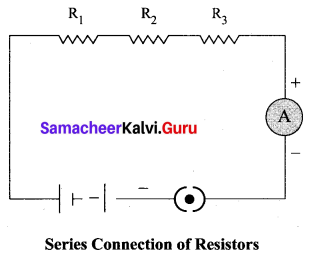(ii) Let, three resistances R1, R2 and R3 be connected in series.

(iii) Let the current flowing through them be I.

(iv) According to Ohm’s Law, the potential differences V1, V2 and V3 across R1 , R2 and R3 respectively, are given by:
V1 = IR1 …. (1)
V2 = IR2 …. (2)
V3 = IR3 …. (3)
The sum of the potential differences across the ends of each resistor is given by:
V = V1 + V2 + V3
using equations (1), (2) and (3), we get
V = IR1 + IR2 + IR3 …. (4)

(v) The effective resistor is a single resistor, which can replace the resistors effectively, so as to allow the same current through the electric circuit.

(vi) Let, the effective resistance of the series-combination of the resistors, be RS. Then,
V = IRS ….(5)
Combining equations (4) and (5)
IRS = IR1 + IR2 + IR3
RS = R1 + R2 + R3
Thus, we can understand that

(vii) When a number of resistors are connected in series, their equivalent resistance or effective resistance is equal to the sum of the individual resistances.

(viii) When V resistors of equal resistance R are connected in series, the equivalent resistance is ‘nR’. i.e., R= nR

(ix) The equivalent resistance in a series combination is greater than the highest of the individual resistances.

(b) Resistors in Parallel:
A parallel circuit has two or more loops through which current can pass. If the circuit is disconnected in one of the loops, the current can still pass through the other loop(s). The wiring in a house consists of parallel circuits.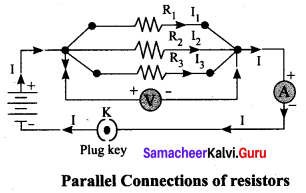(i) Consider that three resistors R1, R2 and R3 are connected across two common points A and B.

(ii) The potential difference across each resistance is the same and equal to the potential difference between A and B. This is measured using the voltmeter.

(iii) The current I arriving at A divides into three branches I1, I2 and I3 passing through R1, R2 and R3 respectively.
According to the Ohm’s law, we have,
I1=VR1 …(1)
I2=VR2 ….(2)
I3=VR3 …. (3)
The total current through the circuit is given by
I = I1 + I2 + I3
Using equations (1), (2) and (3), we get
I=VR1+VR2+VR3 …. (4)
Let the effective resistance of the parallel combination of resistors be RP Then,
I=VRP …. (5)
Combining equations (4) and (5), we have
VRP=VR1+VR2+VR3
1RP=1R1+1R2+1R3.

(iv) When a number of resistors are connected in parallel, the sum of the reciprocals of the individual resistances is equal to the reciprocal of the effective or equivalent resistance.

(v) When ‘n’ resistors of equal resistances R are connected in parallel, the equivalent resistance is Rn
1RP=1R+1R+1R…+1R=nR
Hence, RP=Rn

(vi) The equivalent resistance in a parallel combination is less than the lowest of the individual resistances.

Question 2.
(a) What is meant by electric current?
(b) Name and define its unit.
(c) Which instrument is used to measure the electric current? How should it be connected in a circuit?
(a) Electric current is the rate of flow of charges in a conductor.
(b) The unit of current is ampere. The current flowing through a conductor is said to be one ampere, when a charge of one coulomb flows across any cross-section of a conductor, in one second. Hence,
1 ampere = 1coulomb1second
(c) Ammeter is used to measure electric current. Ammeter is always connected in series.

Question 3.
(a) State Joule’s law of heating.
(b) An alloy of nickel and chromium is used as the heating element. Why?
(c) How does a fuse wire protect electrical appliances?
(a) The heat produced in the resistor is H = W = VQ
We know that the relation between the charge and current is Q = It.
Using this, we get H = VIt
From Ohm’s Law, V = IR. Hence, we have H = I2Rt
This is known as Joule’s law of heating.
Joule’s law of heating states that the heat produced in any resistor is:

• Directly proportional to the square of the current passing through the resistor. H = VIt
• Directly proportional to the resistance of the resistor. V = IR
• Directly proportional to the time for which the current is passing through the resistor. H = I2Rt

(b) An alloy of nickel and chromium is used as the heating element. Because:

• it has high resistivity
• it has a high melting point
• it is not easily oxidized.

(c) (i) The fuse wire is connected in series, in an electric circuit.
(ii) When a large current passes through the circuit, the fuse wire melts due to Joule’s heating effect and hence the circuit gets disconnected.
(iii) Therefore, the circuit and the electric appliances are saved from any damage.
(iv) The fuse wire is made up of a material whose melting point is relatively low.

Question 4.
Explain about domestic electric circuits, (circuit diagram not required)
In our homes, electricity is distributed through the domestic electric circuits wired by the electricians. The first stage of the domestic circuit is to bring the power supply to the main-box from a distribution panel, such as a transformer. The important components of the main-box are: (i) a fuse box and (ii) a meter. The meter is used to record the consumption of electrical energy. The fuse box contains either a fuse wire or a Miniature Circuit Breaker (MCB). The function of the fuse wire or an MCB is to protect the household electrical appliances from overloading due to excess current.

An MCB is a switching device, which can be activated automatically as well as manually. It has a spring attached to the switch, which is attracted by an electromagnet when an excess current passes through the circuit. Hence, the circuit is broken and the protection of the appliance is ensured.

The electricity is brought to houses by one wire has a red insulation and is called the ‘live wire’. The other wire has a black insulation and is called the ‘neutral wire’. The electricity supplied to your house is actually an alternating current having an electric potential of 220 V. Both, the live wire and the neutral wire enter into a box where the main fuse is connected with the live wire. After the electricity meter, these wires enter into the main switch, which is used to discontinue the electricity supply whenever required. After the main switch, these wires are connected to live wires of two separate circuits.

Out of these two circuits, one circuit is of a 5 A rating, which is used to run the electric appliances with a lower power rating, such as tube lights, bulbs and fans. The other circuit is of a 15 A rating, which is used to two insulated wires. Out of these two wires, run electric appliances with a high power rating, such as air-conditioners, refrigerators, electric iron and heaters. It should be noted that all the circuits in a house are connected in parallel, so that the disconnection of one circuit does not affect the other circuit. One more advantage of the parallel connection of circuits is that each electric appliance gets an equal voltage.

Question 5.
(a) What are the advantages of LED TV on normal TV?
(b) List the merits of the LED bulb.
(a) (i) It has a brighter picture quality.
(ii) It is thinner in size.
(iii) It uses less power and consumes very less energy.
(iv) Its life span is more
(v) It is more reliable

(b) (i) As there is no filament, there is no loss of energy in the form of heat. It is cooler than the incandescent bulb.
(ii) In comparison with the fluorescent light, the LED bulbs have a significantly low power requirement.
(iii) It is not harmful to the environment.
(iv) A wide range of colours is possible here.
(v) It is cost-efficient and energy-efficient.
(vi) Mercury and other toxic materials are not required.

IX. Numerical Problems:

Question 1.
An electric iron consumes energy at the rate of 420 W when heating is at the maximum rate and 180 W when heating is at the minimum rate. The applied voltage is 220 V. What is the current in each case?
Solution:
Case: 1
Power (P) = 420W
Applied Voltage (V) = 220V
Current I=PV=420220=1.9A

Case: 2
Power (P) = 180 W
Applied Voltage (V) = 2.20 V
Current I=PV=180220=0.8A

Question 2.
A 100-watt electric bulb is used for 5 hours daily and four 60 watt bulbs are used for 5 hours daily. Calculate the energy consumed (in kWh) in the month of January.
Energy used by 100 W bulb is E = P × t
= 100 × 5 = 500 Wh
Energy used by four 60 W bulbs E = 4 × 60 × 5 = 1200 Wh
Total energy per day = 500 + 1200 = 1700 Wh
= 1.7 kWh
Number of days in January = 31 days.
Energy consumed in January = 31 × 1.7 = 52.7 kWh.

Question 3.
A torch bulb is rated at 3 V and 600 mA. Calculate it’s?
(a) power
(b) resistance
(c) energy consumed if it is used for 4 hours.
Solution:
V = 3V and I = 600 mA = 600 × 10-3 A
(a) Power (P) = VI
= 3 × 600 × 10-3
= 1800 × 10-3
= 1.8 W (or) watt.

(b) Resistance (R) = VI = 3600×10−3 = 5 Ω.

(c) Power (P) = 1.8 W and
Time = 4 hours = 4 × 60 × 60 = 14400 second
Energy consumed E = P × t = 1.8 × 14400 = 25920 joules
E = 25.9 KJ.

Question 4.
A piece of wire having a resistance R is cut into five equal parts.
(a) How will the resistance of each part of the wire change compare with the original resistance?
(b) If the five parts of the wire are placed in parallel, how will the resistance of the combination change?
(c) What will be the ratio of the effective resistance in series connection to that of the parallel connection?
Solution:
(a) Consider a piece of wire having resistance R. It cut into 5 equal parts. So number of equal resistros are 5. n = 5
When ‘n’ resistors of equal resistance R are connected in series, the equivalent resistance is ‘nR’
RS = nR
⇒ RS = 5R
⇒ R = Rs5
⇒ R = 0.2 RS
Each part of resistance ‘R’ is equal to 0.2 times of original resistance.

(b) Effective Resistance of 5 Resistors
1RP=1R+1R+1R+1R+1R = 5R
RP=R5=0.2R = 0.2 R.

(c) Effective resistance of series combination RS = 5R
Effective resistance of parallel combination Rp=R5
The ratio of series connection to the parallel connection
RsRp=5R(R/s)
RsRp=5R×5R = 25
RS : RP = 25 : 1.

XI. HOTS Questions

Question 1.
Two resistors when connected in parallel give the resultant resistance of 2 ohms, but when connected in series the effective resistance becomes 9 ohms. Calculate the value of each resistance.
Solution:
Resultant resistance of parallel combination RP = 2 Ω
Resultant resistance of series combination RS = 9 Ω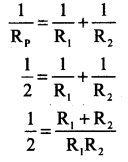2(R1 + R2) = R1R2 ….. (1)
RS = R1 + R2
⇒ 9 = R1 + R2
⇒ R1 = 9 – R2 ….. (2)
Substitute equation (2) in equation (1)
2(9 – R2 + R2) = (9 – R2) R2
⇒ 18=9R2−R22
⇒ R22−9R2+18=0
⇒ (R2 – 3) (R2 – 6) = 0
⇒ R2 = 3, 6
(i) If R2 = 3; R1 = 9 – R2 = 9 – 3 = 6 Ω
(ii) If R2 = 6; R1 = 9 – R2 = 9 – 6 = 3 Ω.

Question 2.
How many electrons are passing per second in a circuit in which there is a current of 5 A?
A current 1018 electrons
A current of 5A consists
= 5 × 6.25 × 1018 electrons = 31.25 × 1018
Number of electrons passing per second
= 31.25 × 1018 electrons

Question 3.
A piece of wire of resistance 10 ohms is drawn out so that its length is increased to three times its original length. Calculate the new resistance.
Solution:
Specific resistance (ρ) = RAl
R=ρlA
When the length is increased by three and then the area of cross-section is reduced by three,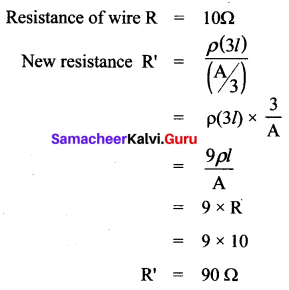### Samacheer Kalvi 10th Science Electricity Additional Questions

Question 1.
The motion of electric charges through a conductor will constitute ______.
(a) electric current
(b) electric potential
(c) electric field
(d) none.
(a) electric current

Question 2.
Electric charge is expressed in:
(a) Volt
(b) Joule
(c) Coulomb
(d) Ohm
(c) Coulomb

Question 3.
A charge of 60 coulomb flows through a bulb in 5 minutes what is the current through the bulb?
(a) 2 A
(b) 0.2 A
(c) 12 A
(d) 0.12 A.
(b) 0.2 A
Hint: I=qt=605×60=15=0.2A

Question 4.
1 coulomb of charge is equivalent to the charge of:
(a) 6.25 × 1018 electrons
(b) 6 × 1018 protons
(c) 1.6 × 10-19 electrons
(d) 1.6 × 10-19 protons
(a) 6.25 × 1018 electrons

Question 5.
The S.I. unit of electric potential difference is ______.
(a) Ampere
(b) Joule
(c) Watt
(d) Volt.
(d) Volt

Question 6.
The potential difference V is proportional to the current I, the graph between V and I is a _____.
(a) straight line
(b) parabola
(c) ellipse
(d) none.
(a) straight line

Question 7.
In an electric circuit, voltmeter reads 24V and ammeter reads 6A. The value of resistance is:
(a) 40Ω
(b) 2Ω
(c) 0.25Ω
(d) 4Ω
(d) 4Ω

Question 8.
What is the potential difference between the ends of a resistor of 15 Ω when a current of 2 A passes through it?
(a) 30 V
(b) 7.5 V
(c) 3 V
(d) 300 V.
(a) 30 V

Question 9.
How much heat is generated when current I is passing through a resistor for time t?
(a) I2Rt
(b) IR2t
(c) VI
(d) I2R
(a) I2Rt

Question 10.
The unit of conductance is _____.
(a) ohm-1
(b) volt-1 ampere
(c) both (a) and (b)
(d) ohm.
(c) both (a) and (b)

Question 11.
Two electric bulbs have resistances in the ratio 1 : 2. If they are joined in series, the energy consumed in these are in the ratio:
(a) 1 : 2
(b) 2 : 1
(c) 4 : 1
(d) 1 : 1
(a) 1 : 2

Question 12.
The resistance of a wire of length 10 cm is 2 ohm, then its conductance is _____.
(a) 0.5 ohm
(b) 5 ohm-1
(c) 0.5 ohm-1
(d) 20 ohm-1.
(c) 0.5 ohm-1

Question 13.
The value of current flowing through a circuit consisting of two resistance 6Ω and 18Ω in series with a battery of 3 V is:
(a) 0.5 A
(b) 0.125 A
(c) 6 A
(d) 0.25 A
(b) 0.125 A

Question 14.
When two 2 Ω resistors are connected in parallel, the effective resistance is _____.
(a) 4 Ω
(b) 1 Ω
(c) 0.5 Ω
(d) 5 Ω.
(b) 1 Ω

Question 15.
When two 2 Ω resistors are connected in series, the effective resistance is ______.
(a) 1 Ω
(b) 4 Ω
(c) 5 Ω
(d) 2 Ω.
(b) 4 Ω

Question 16.
One kilowatt-hour is:
(a) 3.6 × 106J
(b) 1000 W
(c) 3600 W-1
(d) 2.778 × 103 J
(a) 3.6 × 106J

Question 17.
When ‘n’ number of resistors are connected in parallel, the effecive resistance for parallel is _____.
(a) nR
(b) nR
(c) Rn
(d) none of these.
(c) Rn

Question 18.
The effective resistance for the given circuit in AB _____.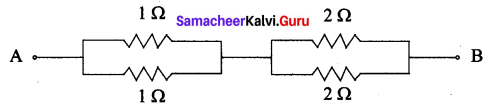(a) 1 Ω
(b) 2 Ω
(c) 3 Ω
(d) 1.5 Ω.
(d) 1.5 Ω.

Question 19.
The effective resistance between AB in the given circuit _____.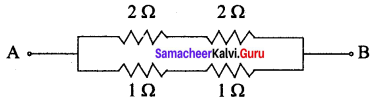(a) 14 Ω
(b) 12 Ω
(c) 34 Ω
(d) 43 Ω.
(d) 43 Ω.

Question 20.
The symbol of Ammeter is:
(a) V
(b) A
(c) G
(d) I
(b) A

Question 21.
Tungsten material is used in _____.
(a) Fuse wire
(b) bulbs
(c) batteries
(d) none.
(b) bulbs

Question 22.
When a charge 24 C flow through a bulb in 10 second then the current flowing through the bulb is:
(a) 1.2 A
(b) 2.4 A
(c) 4.8 A
(d) 0.06 A
(b) 2.4 A

Question 23.
The unit of electric power is _____.
(a) Volt-ampere
(b) Watt
(c) both (a) and (b)
(d) only (b).
(c) both (a) and (b)

Question 24.
One horsepower is equal to _____.
(a) 764 watt
(b) 746 watt
(c) 647 watt
(d) 674 watt.
(b) 746 watt

Question 25.
Unit of specific resistance is:
(a) mho
(b) mho m
(c) ohm m
(d) ampere
(c) ohm m

Question 26.
To protect the horse hold electrical appliances from overloading due to excess current _____.
(a) Fuse wire
(b) MCB
(c) both (a) and (b)
(d) none.
(c) both (a) and (b)

Question 27.
In India, domestic circuits are supplied with a frequency of ______.
(a) 60 Hz
(b) 50 Hz
(c) 220 Hz
(d) 230 Hz.
(b) 50 Hz

Question 28.
What is the unit of conductivity?
(a) ohm m-1
(b) mho m
(c) ohm m
(d) mho m-1
(d) mho m-1

Question 29.
The colour of the LED will depend on the type of _____ used.
(a) circuit
(b) materials
(c) display
(d) segment.
(b) materials

Question 30.
One way of overcoming the energy crisis is to use more _______ bulbs.
(a) filament
(b) glass
(c) LCD
(d) LED.
(d) LED

Question 31.
When resistances are connected in parallel current is more as effective resistance is:
(a) maximum
(b) less
(c) more
(d) minimum
(b) less

Question 32.
The current in the electric bulb of 100 W and 200 V electric circuit is _____.
(a) 5 A
(b) 0.5 A
(c) 50 A
(d) 500 A.
(b) 0.5 A

II. Fill in the Blanks

Question 1.
Rate of flow of charges in a conductor is _____.
electric current.

Question 2.
_____ is used to fix the magnitude of the current through a circuit.
Resistor.

Question 3.
______ is used to select the magnitude of the current through a circuit.
Rheostat.

Question 4.
_____ is used to measure the current.
Ammeter.

Question 5.
______ is used to measure the potential difference.
Voltmeter.

Question 6.
______ is used to indicate the direction of the current.
Galvanometer.

Question 7.
Electric current passes in the circuit from _____ terminal to the _____ terminal.
positive, negative.

Question 8.
Electrical resistivity is _____ for different materials.
different.

Question 9.
Reciprocal of resistance is ______.
conductance.

Question 10.
Reciprocal of electrical resistivity is ______.
electrical conductivity.

Question 11.
The equivalent resistance in a series combination is _____ than the highest of the individual resistances.
greater.

Question 12.
The equivalent resistance in a parallel combination is _____ than the lowest of the individual resistances.
less.

Question 13.
The heating effect of current is used in devices like _____ and _____.
electric heater, electric iron.

Question 14.
Nichrome is an alloy of _____ and _____.
Nickel, Chromium.

Question 15.
The filament is made up of a material whose ______ is very high.
melting point.

Question 16.
Electric power is the product of _____ and _____.
electric current, a potential difference.

Question 17.
The important components of main box are _____ and _____.
fuse box, meter.

Question 18.
LED is _____.
Light Emitting Diode.

Question 19.
LCD is _____.
Liquid Crystal Display.

Question 20.
An array of LEDs act as ______.
pixels.

Question 21.
A _____ is the display device used to give an output in the form of _____.
Seven Segment display, numbers or text.

III. Match the following.

Question 1.

 1. LED (a) heating device 2. Fuse wire (b) alloy 3. Tungsten (c) semiconductor device 4. Nichrome (d) Filament 5. Electric heater (e) MCB

1. (c) semiconductor device
2. (e) MCB
3. (d) Filament
4. (b) alloy
5. (a) heating device

Question 2.

 1. Power (a) V = IR 2. Joule’s law (b) ρ=RAl 3. Ohm’s law (c) P = VI 4. Specific Resistance (d) I = Qt 5. Electric energy (e) H = I2RT

1. (c) P = VI
2. (e) H = I2RT
3. (a) V = IR
4. (b) ρ=RAl
5. (e) I = Qt

Question 3.

 1. Ammeter (a) To measure resistance 2. Voltmeter (b) Direction of current 3. Galvanometer (c) To measure voltage 4. Ohmmeter (d) To measure current

1. (d) To measure current
2. (c) To measure voltage
3. (b) Direction of current
4. (a) To measure resistance

Question 4.

 1. ρ=RAl (a) watt 2. σ=1ρ (b) Ωm 3. E = P × t (c) coulomb 4. P = VI (d) m-1Ω-1 5. q = It (e) Kilowatt-hour

1. (b) Ω-1m
2. (d) m-1-1
3. (e) Kilowatt-hour
4. (a) watt
5. (c) coulomb

Question 5.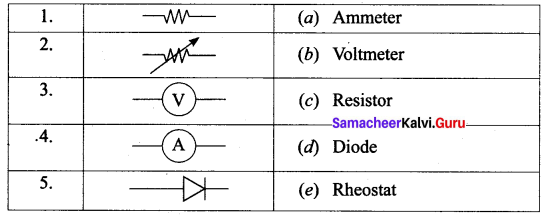1. (c) Resistor
2. (e) Rheostat
3. (b) Voltmeter
4. (a) Ammeter
5. (d) Diode

IV. State whether the following statements are true or false: If false correct the statement.

Question 1.
The equivalent resistance in a series combination in lesser than the highest of the individual resistances.
False.
Correct Statement: The equivalent resistance in a series combination is greater than the highest of the individual resistances.

Question 2.
When ‘n’ number of resistors of equal resistance R connected in series, the equivalent resistance is RP=Rn.
False.
Correct Statement: RS = nR.

Question 3.
When resistors are connected in series, a current is less as effective resistance is more.
False.
Correct Statement: Current is more as effective resistance is less.

Question 4.
Nichrome is an alloy of copper and chromium.
False.
Correct Statement: Nichrome is an alloy of nickel and chromium.

Question 5.
The fuse wire is made up of a material whose melting point is relatively high.
False.
Correct Statement: The fuse wire is made up of a material whose melting point is relatively low.

Question 1.
When a steady current flows in a conductor of non-uniform cross-section will the charge passing per unit time depend on area of cross section of the conductor?
No, the charge passing per unit time through the conductor is independent of area of cross-section of the wire.

Question 2.
Name any two – component and give its use?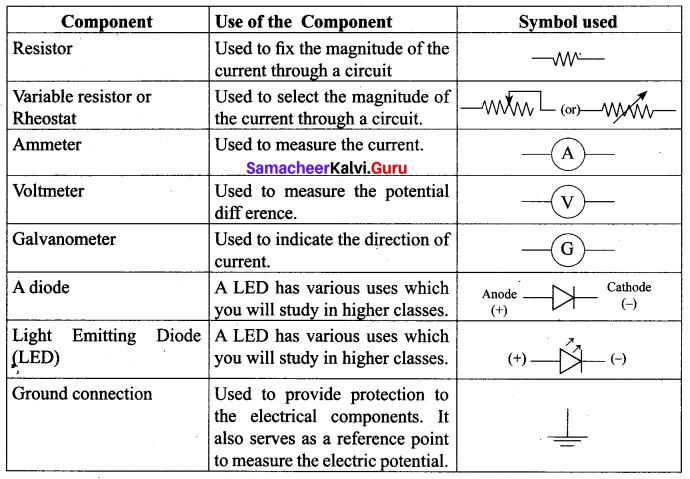Question 3.
Define the unit of electric potential?

1. The SI unit of electric potential or potential difference is volt (V).
2. The potential difference between two points is one volt if one joule of work is done in moving one coulomb of charge from one point to another against the electric force.
1 volt =1 joule 1 coulomb

Question 4.
Draw a graph between V and I for a conductor by ohm’s law?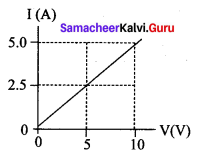Question 5.
What is the relationship between conductance and resistance? State the Unit of conductance.
conductance = 1resistance
G = 1R
Unit of conductance is mho.

Question 6.
Define the unit of electric power.
The SI unit of electric power is watt. When a current of 1 ampere passes across the ends of a conductor, which is at a potential difference of 1 volt, then the electric power is
P = 1 volt × 1 ampere = 1 watt.

Question 7.
Define one kilowatt-hour? Give its value?
One kilowatt-hour is otherwise known as one unit of electrical energy. One kilowatt-hour means that electric power of 1000 watt has been utilized for an hour.
1 kWh = 1000 watt-hour = 1000 × (60 × 60) watt-second = 3.6 × 106 J.

Question 8.
Define electric current.
Electric current is defined as the rate of flow of charge in a conductor.

Question 1.
Define electrical resistivity? Give its unit.
The electrical resistivity of a material is defined as the resistance of a conductor of unit length and unit area of cross – section.
Its unit is ohm metre.

Question 2.
Define specific resistance electrical conductivity? Give its unit.
The reciprocal of electrical resistivity of a material is called its electrical conductivity.
σ=1ρ
Its unit is ohm-1 metre-1. It is also represented as mho metre-1.

Question 3.
Differences between series and parallel circuit?

 Criteria Series Parallel Equivalent resistance More than the highest resistance Less than the lowest resistance Amount of current Current is less as effective resistance is more Current is more as effective resistance is less Switching ON/OFF If one appliance is disconnected, others also do not work. If one appliance is disconnected, others will work independently.

Question 4.
Distinguish electric power and electric energy?

 Electric Power Electric Energy Electric Power is defined as the rate of doing work It is the capacity to do electric work P = VI E = P × t Unit is watt Unit is joule (or) [kWh]

Question 5.
Define electric potential difference.
The electric potential difference between two points is defined as the amount of work done in moving a unit positive charge from one point to another point against the electric force.

Question 6.
Define Volt.
The potential difference between two points is one volt, if one joule of work is done in moving one coulomb of charge from one point to another against the electric force.
1 volt = 1joule1coulomb

Question 7.
What is the unit of resistance? Define its unit of resistance is ohm.
Resistance of a conductor is said to be one ohm if a current of one ampere flows through it when a potential difference of one volt is maintained across its ends.
1 ohm = 1volt1amphre

Question 1.
Explain the series connection of parallel resistors.
(i) If we consider the connection of a set of parallel resistors that are connected in series, we get a series – parallel circuit.
(ii) Let R1 and R2 be connected in parallel to give an effective resistance of RP1.
(iii) Similarly, let R3 and R4 be connected in parallel to give an effective resistance of RP2.
(iv) Then, both of these parallel segments are connected in series.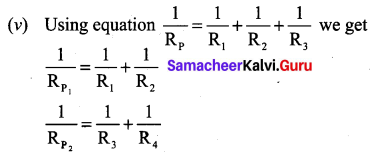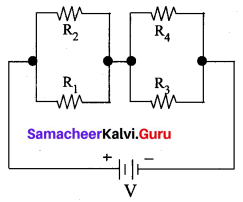Finally, using the equation the RS = R1 + R2 + R3 net effecitve resistance is given by Rtotal = RP1 + RP2

Question 2.
Explain the parallel connection of series resistors.
(i) Let R1 and R2 be connected in parallel to give an effective resistance of RP1.
(ii) If you consider a connection of a set of series resistors connected in a parallel circuit, we get a parallel-series circuit.
(iii) Let R1 and R2 be connected in series to give an effective resistance of RS1.
(iv) Similarly, let R3 and R4 be connected in series to give an effective resistance of RS2.
(v) Then, both of these serial segments are connected in parallel.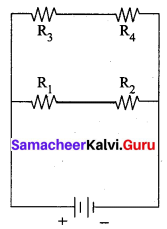Parallel – Series combination of resistors
Using equation RS = R1 + R2 + R3 we get
RS = R1 + R2 + R3
RS1 = R1 +R2
RS2 = R3 + R4
Finally, using equation, 1Rp=1R1+1R2+1R3 the net effective resistance is given by 1Rtotal =1Rs1+1Rs2.

VIII. Numerical Problems

Question 1.
Show that one ampere is equivalent to a flow of 6.25 × 1018 elementary charges per second.
Solution:
I = 1 A, t = 1 s, e = 1.6 × 10-19 C
q = ne
I=qt=net
Number of electrons, n=Ite=1×11.6×10−19=6.25×1018.

Question 2.
How many electrons pass through a lamp in one minute, if the current is 300 mA?
Solution: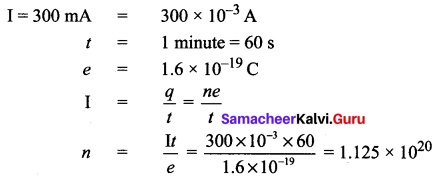Question 3.
Calculate the resistivity of the material of wire 10 m long, 0.4 mm in diameter and having a resistance of 20 Ω.
Solution:
l = 10 m; d = 0.4 mm = 0.4 × 10-3 m
r = 0.2 × 10-3 m
R = 20 Ω
Resistivity (or) specific resistance of a material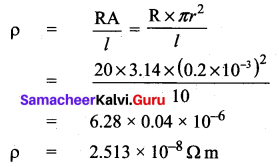Question 4.
Find the effective resistance between A and B in the given circuit.
Solution:
Effective resistance in parallel combination 3 Ω and 2 Ω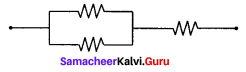1Rp=1R1+1R2=13+12=2+36=56Ω
RP=65 = 1.2 Ω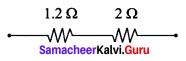RS = R1 + R2 = 1.2 + 2 = 3.2 Ω.

## How to Prepare using Samacheer Kalvi 10th Science Chapter 4 Electricity Notes PDF?

Students must prepare for the upcoming exams from Samacheer Kalvi 10th Science Chapter 4 Electricity Notes PDF by following certain essential steps which are provided below.

• Use Samacheer Kalvi 10th Science Chapter 4 Electricity notes by paying attention to facts and ideas.
• Pay attention to the important topics
• Refer TN Board books as well as the books recommended.
• Correctly follow the notes to reduce the number of questions being answered in the exam incorrectly
• Highlight and explain the concepts in details.

## Frequently Asked Questions on Samacheer Kalvi 10th Science Chapter 4 Electricity Notes

#### How to use Samacheer Kalvi 10th Science Chapter 4 Electricity Notes for preparation??

Read TN Board thoroughly, make separate notes for points you forget, formulae, reactions, diagrams. Highlight important points in the book itself and make use of the space provided in the margin to jot down other important points on the same topic from different sources.

#### How to make notes for Samacheer Kalvi 10th Science Chapter 4 Electricity exam?

Read from hand-made notes prepared after understanding concepts, refrain from replicating from the textbook. Use highlighters for important points. Revise from these notes regularly and formulate your own tricks, shortcuts and mnemonics, mappings etc.
Share: